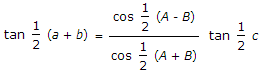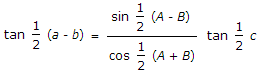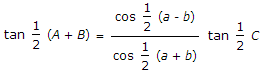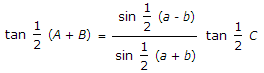# Civil Engineering - Advanced Surveying

### Exercise :: Advanced Surveying - Section 3

46.

23 cm x 23 cm photographs are taken from a flying height with a camera of focal length of 3600 m and 15.23 cm respectively. A parallax difference of 0.01 mm represents

 A. 1 m B. 2 m C. 3 m D. 4 m E. 5 m.

Explanation:

No answer description available for this question. Let us discuss.

47.

For a spherical triangle ABC, pick up incorrect statement

 A.B.C.D.E. none of these.

Explanation:

No answer description available for this question. Let us discuss.

48.

A plate parallel is the line on the plane of the negative

 A. parallel to the principal line B. perpendicular to the principal line C. along the bisector of the angle between the principal line and a perpendicular line through principal plane D. none of these.

Explanation:

No answer description available for this question. Let us discuss.

49.

The great circle which passes through the zenith, nadir and the poles, is known as

 A. meridian B. vertical circle C. prime vertical D. none of these.

Explanation:

No answer description available for this question. Let us discuss.

50.

In triangulation surveys

 A. the area is divided into triangular figures B. control stations are located from which detailed surveys are carried out C. sides are not measured excepting the base line D. angular measurements are only resorted to E. all the above.